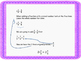# Adding a Mixed Number and a Fraction Mini LessonSubject
Resource Type
File Type
PDF (1 MB|8 pages)
Standards
FREE
• Product Description
• Standards
This is Power Point Presentation showing how to add a mixed number and a fraction.
This presentation explains how students can add the fractional part first and then go back and add in the whole number.
Students are shown how to draw number bonds to make improper fractions into mixed numbers.
Add and subtract mixed numbers with like denominators, e.g., by replacing each mixed number with an equivalent fraction, and/or by using properties of operations and the relationship between addition and subtraction.
Total Pages
8 pages